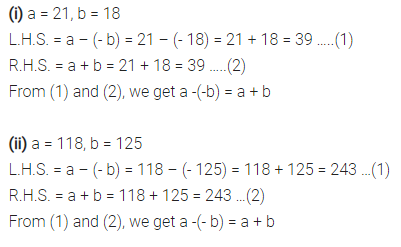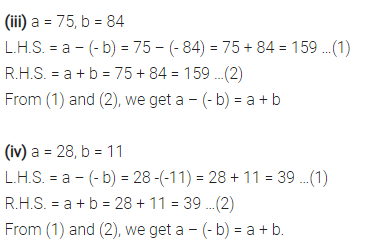# Verify a – (- b) = a + b for the following values of a and b. (i) a = 21, b = 18

Verify a – (- b) = a + b for the following values of a and b.
(i) a = 21, b = 18
(ii) a = 118, b = 125
(iii) a = 75, b = 84
(iv) a = 28, b = 11.